• 二阶线性非齐次微分方程非齐 重要的性质、定理（共6条）： 证明1：若y1、y2、y3是非齐的，a、b、c为常数且a+b+c=0，y=a y1+by2+cy3则y是齐的 由(7) ，则需要证明a y1+by2+cy3是 齐的。 因为y1、...
以下简称
二阶线性齐次微分方程 为 齐二阶线性非齐次微分方程 为 非齐

重要的性质、定理（共6条）：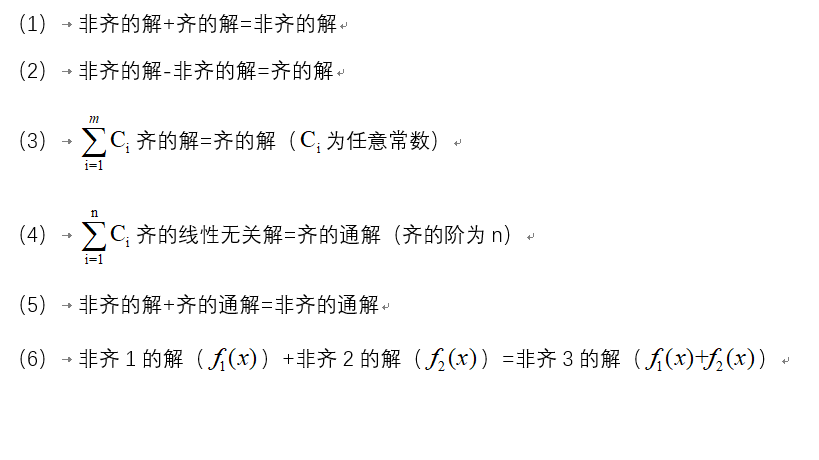证明1：若y1、y2、y3是非齐的解，a、b、c为常数且a+b+c=0，y=a y1+by2+cy3则y是齐的解
由(7) ，则需要证明a y1+by2+cy3是 齐的解。
因为y1、y2、y3是非齐的解，故y1－y2、y2-y3是齐的解，则  C1（y1－y2）+C2（y2-y3）也是齐的解  故C1（y1－y2）+C2（y2-y3）就是齐的解  显然y1的系数=C1=a  y2的系数=-C1+C2=b  y3的系数=-C2=c  a+b+c=0

证明2： 若y1、y2、y3是非齐的解，a、b、c为常数且a+b+c=１，y=a y1+by2+cy3 则y是非齐的解
由（1），则需要证明a y1+by2+cy3是非齐的解＋齐的解
因为y1、y2、y3是非齐的解，故y1－y2、y2-y3是齐的解，则  C1（y1－y2）+C2（y2-y3）也是齐的解，而y3是非齐的解，  故C1（y1－y2）+C2（y2-y3）+y3就是非齐的解  显然y1的系数=C1=a  y2的系数=-C1+C2=b  y3的系数=-C2+1=c  a+b+c=1

证明3：若y1、y2、y3是非齐的线性无关解，a、b、c为任意常数且a+b+c=0，
y=a y1+by2+cy3，则y是齐的通解  由（7）  y1、y2、y3是非齐的线性无关解，现假设y1-y2、y2-y3也是线性无关的，  则齐的通解y=C1(y1-y2)+C2(y2-y3)  显然y1的系数=C1=a  y2的系数=-C1+C2=b  y3的系数=-C2=c  a+b+c=0
补充证明：现证明y1-y2、y2-y3是线性无关的。
假设线性有关，则存在不全为0的k1和k2，  使得k1（y1-y2）+k2（y2-y3）恒等于0  即：  (k1)y1+(k2-k1)y2-(k2)y3恒等于0  由于y1、y2、y3是线性无关的，故k1=0、k2=0，与假设相违背。因此，y1-y2、y2-y3是线性无关的。

证明4：若y1、y2、y3是非齐的线性无关解，a、b、c为任意常数且a+b+c=1，y=a y1+by2+cy3，则y是非齐的通解
由（5）  y1、y2、y3是非齐的线性无关解，现假设y1-y2、y2-y3也是线性无关的，  则齐的通解y=C1(y1-y2)+C2(y2-y3) ，  非齐的解为y3（你换成y1或者y2都是一样的，因为最后大家系数都是要加在一起的）  显然y1的系数=C1=a  y2的系数=-C1+C2=b  y3的系数=-C2+1=c  a+b+c=1
展开全文• 二阶常系数非齐次线性微分方程的通解 二阶常系数非齐次线性微分方程的...二阶常系数齐次线性微分方程通解的解法：二阶常系数齐次线性微分方程的通解 下面只需要解出微分方程的特解即可 对应微分方程： ay...
二阶常系数非齐次线性微分方程的通解
见课文原文：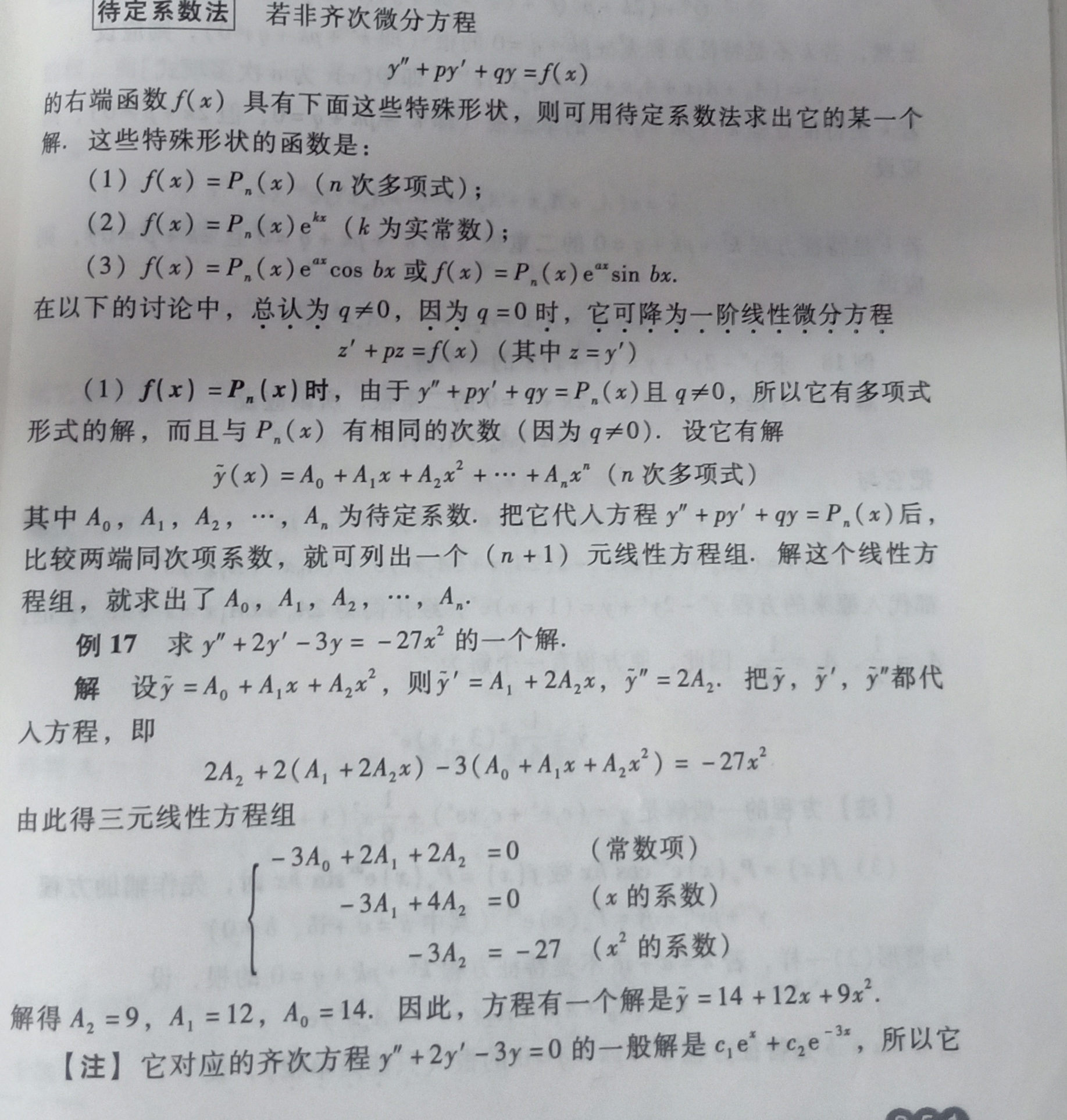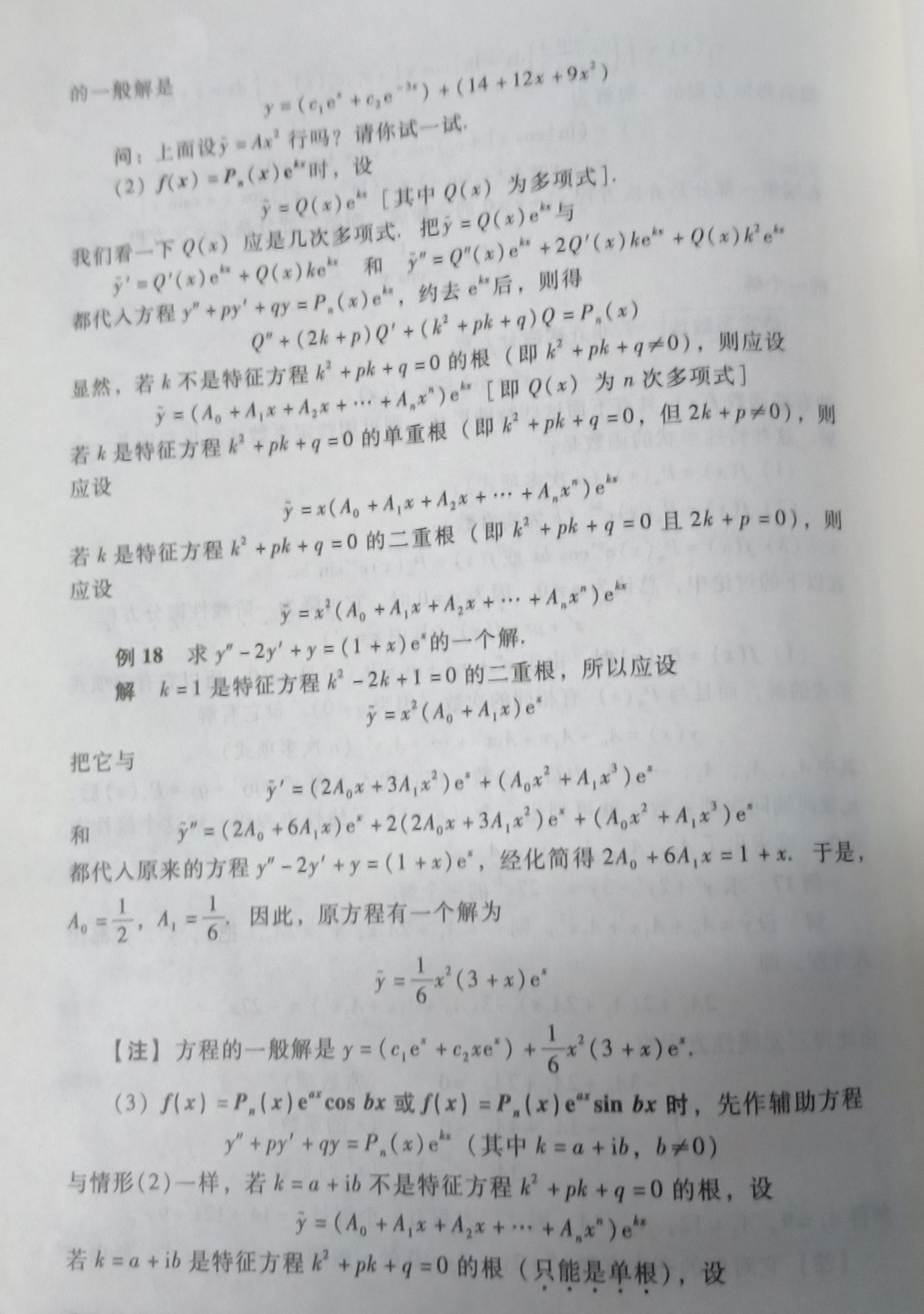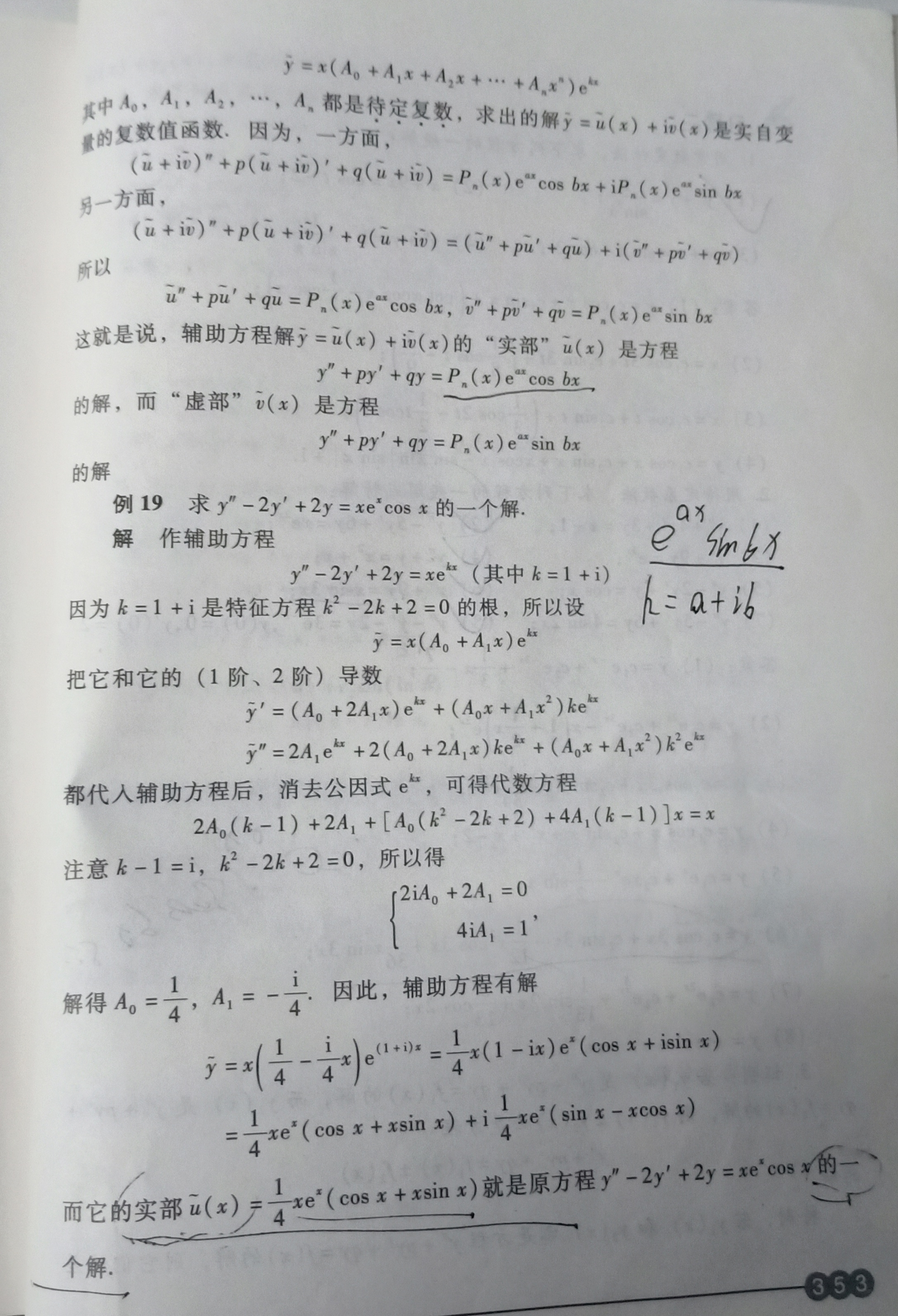下面看转的一片博客文章：
二阶常系数非齐次线性微分方程的形式为：
							    ay″+by′+cy=f(x)

微分方程的通解 = 对应的二阶常系数齐次线性微分方程通解 + 自身的一个特解 简单记为：通解 = 齐次通解 + 特解。
二阶常系数齐次线性微分方程通解的解法：二阶常系数齐次线性微分方程的通解
下面只需要解出微分方程的特解即可
对应微分方程：
							    ay″+by′+cy=f(x)

右式f(x)有两种形式： ①f(x)=

e

λ

x

P

m

(

x

)

e^{\lambda x}Pm(x)

型 此时微分方程对应的特解为： y∗=xkRm(x)eλx
其中：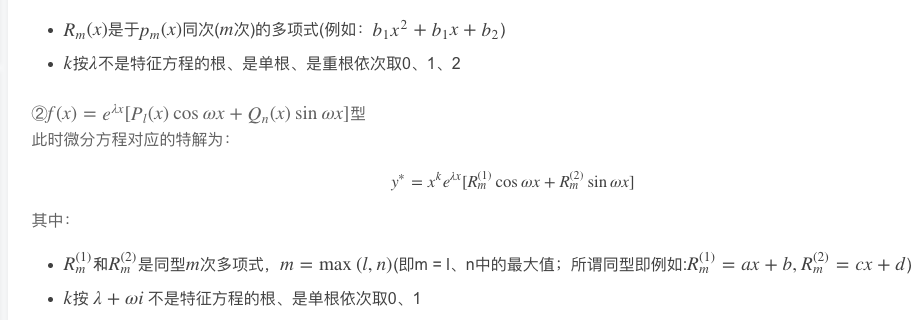得到这个不完全的特解后根据需要求出其不同阶的导数然后带入微分方程，即可解出特解中的系数，到这里，就得到了微分方程的完整特解，于齐次通解相加即的微分方程的通解。
例： 求微分方程 2y″+y′−y=2

e

x

e^{x}

的通解
解： 微分方程对应的齐次微分方程的特征方程为 2

r

2

r^{2}

+r−1=0 可得通解：

y

=

c

1

e

−

x

+

c

2

e

1

2

x

y=c^{_{1}}e^{-x}+c^{_{2}}e^{\frac{1}{2}x}

微分方程的右式f(x)=2e^x满足f(x)=

e

λ

x

e^{\lambda x}

Pm(x)型，且λ=1,m=0λ=1,m=0， 所以，设特解为：
y∗=a

e

x

e^{x}

所以y∗=a

e

x

e^{x}

、y∗′=a

e

x

e^{x}

、y∗″=a

e

x

e^{x}

带入微分方程左式得：2a

e

x

+

a

e

x

−

a

e

x

e^{x}+ae^{x}−ae^{x}

=2e^{x}
得：a=1
所以特解为：
y∗=

e

x

e^{x}

微分方程的通解为：

y

=

c

1

e

−

x

+

c

2

e

1

2

x

+

e

x

y=c^{_{1}}e^{-x}+c^{_{2}}e^{\frac{1}{2}x}+e^{x}

转自：https://blog.csdn.net/baishuiniyaonulia/article/details/79690752
展开全文• 一般二阶线性非齐次微分方程与对应齐次方程的关系@(微积分)设p(x),q(x),f(x),f(x)≠0p(x),q(x),f(x),f(x)\neq 0为连续函数，对于下面的二阶线性非齐次方程：y″+p(x)y′+q(x)y=f(x)y''+p(x)y'+q(x)y = f(x) ...
一般二阶线性非齐次微分方程的解与对应齐次方程的解的关系
@(微积分)
设

p(x),q(x),f(x),f(x)≠0
$p(x),q(x),f(x),f(x)\neq 0$为连续函数，对于下面的二阶线性非齐次方程：

y″+p(x)y′+q(x)y=f(x)
$y''+p(x)y'+q(x)y = f(x)$ (1)
对应的二阶线性齐次方程：

y″+p(x)y′+q(x)y=0
$y''+p(x)y'+q(x)y = 0$ (2)
有下面的论断：

y1(x),y2(x),y3(x)
$y_1(x),y_2(x),y_3(x)$是(1)的三个解，a,b,c是三个常数，并设：

y=ay1(x)+by2(x)+cy3(x)
$y = ay_1(x)+by_2(x)+cy_3(x)$,
那么y是(1)的解的充要条件是：a+b+c = 1y是(2)的解的充要条件是a+b+c=0

y1(x),y2(x),y3(x)
$y_1(x),y_2(x),y_3(x)$是(1)的三个线性无关的解，a,b,c是**两个任意常数，并设：

y=ay1(x)+by2(x)+cy3(x)
$y = ay_1(x)+by_2(x)+cy_3(x)$,
那么y是(1)的通解的充要条件是：a+b+c = 1y是(2)的通解的充要条件是a+b+c=0
思考一道题目：

设

p(x),q(x),f(x)
$p(x),q(x),f(x)$均是x的已知连续函数，

y1(x),y2(x),y3(x)
$y_1(x),y_2(x),y_3(x)$是

y″+p(x)y′+q(x)y=f(x)
$y''+p(x)y'+q(x)y = f(x)$的三个线性无关的解，

C1,C2
$C_1,C_2$是两个任意常数，则该非齐次线性微分方程的通解是：C

A.(C1+C2)y1+(C2−C1)y2+(1−C2)y3
$A. (C_1+C_2)y_1+(C_2-C_1)y_2+(1-C_2)y_3$

B.(C1+C2)y1+(C2−C1)y2+(C1−C2)y3
$B. (C_1+C_2)y_1+(C_2-C_1)y_2+(C_1-C_2)y_3$

C.C1y1+(C2−C1)y2+(1−C2)y3
$C. C_1y_1+(C_2-C_1)y_2+(1-C_2)y_3$

D.C1y1+(C2−C1)y2+(C1−C2)y3
$D. C_1y_1+(C_2-C_1)y_2+(C_1-C_2)y_3$

直接利用上面的定理可知：系数之和为1时，即为通解。那么检验可得C是满足的。
展开全文• 一，二阶非齐次线性ODE的标准形式： 表示输入、驱动  表示输出、响应 二，通解：   叫补充解，是齐次方程通解 （作为的相伴方程） 是非齐次方程的特解 三，应用： 弹簧—质量—阻尼系统（第九讲） 如...
一，二阶非齐次线性ODE的标准形式：
表示输入、驱动 表示输出、响应
二，通解：
叫补充解，是齐次方程的通解（作为的相伴方程）是非齐次方程的特解
三，应用：
弹簧—质量—阻尼系统（第九讲）如图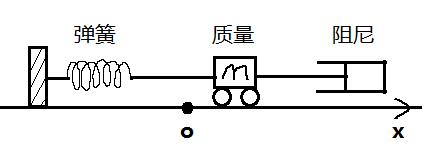在系统中，对小车施加额外的一个力标准形式：被动系统：当时，系统没有外力干涉，只是被动地对初始条件进行响应受迫系统：当时，系统一直有外力干涉，强迫它运动

电路（第八讲）如图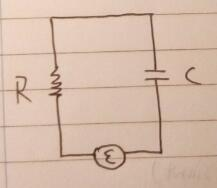R表示电阻，C表示电容，q表示电荷，j表示电流，表示电动势在电路中，串联额外的一个电感L基尔霍夫定律：当环绕电路运行时，元件的电压降之和为0数学模型：，两边求导：被动电路：当时，比如电动势是干电池，或者电路中没有电源，电路中的电荷会趋于静止受迫电路：当时，比如电动势是交流电源，强迫电荷运动
四，证明通解：
将化为L表示二阶线性算子证明1：是解
将代入原方程：，
证明2：是所有的解
取任意一个方程的解齐次方程的解：和是所有常数中选出的特定值
五，区分稳态解和暂态解的条件：
一阶常系数非齐次线性ODE：，k为常数通解：当时，是齐次方程，是它的通解（是的相伴方程）非齐次方程的特解当时，为稳态解，为暂态解（见第三讲第七点）当时，，无法区分

二阶常系数非齐次线性ODE：，A和B是常数通解：当所有特征根都具有负实部时，为稳态解，为暂态解，如下表：
特征根相伴方程的通解通解趋于0的条件，  
展开全文笔记
• *本文略去了很多证明，只记录结论 *文中的微分方程均指代二阶常...由于是二阶线性微分方程，所以它有两个，记为y1、y2y1、y2y_1、y_2，若y1y2≠Cy1y2≠C\frac{y_1}{y_2} \neq C(即两个之比不为常数)，则y1、...高等数学
• 介绍了求解二阶常系数非齐次线性微分方程的2种简易方法———降阶法和积分法,扩大了可求解二阶常系数非齐次线性微分方程的范围,并举例说明了它们的应用.
• 在假设二阶变系数非齐次线性微分方程两个变系数关系已知的前提下，利用降阶法推出几类二阶变系数齐次线性微分方程通解表达式.
• 人生有无数的可能性，考研的结果一定不是终点!但做的每一个选择都要坚持到最后!这是对自己、对梦想最大的尊重!用探索方法代替消极迷茫，用寻求技巧抵消杂乱慌张!争分夺秒，竭尽所能!悉心浇灌，静候花开!...数学
• 二阶常系数线性方程通解反推方程@(微积分)引例是这样的： 设cosxcosx与xexxe^x为某n阶常系数线性齐次方程的两个解，则最小的n = ？，相应的首项系数为1的方程是？ 分析：由cosx是一个解，则必有另一解sinx,±i\...
• 不要嘲笑我，我百度经验搬过来的二阶常系数线性非齐次方程的形式如下2基本求解思路如下，我们先要有一个总的思路用于解题3难点：对于特的求法END一.特求法一：待定系数法1优点：简单易懂，不易错缺点：计算量...
• :证明二阶齐次线性方程通解可以表示成任意两个线性无 86 关解的线性组合}} \setlength\epigraphwidth{ 0.7 \linewidth} 87 \epigraph{汝当更求古之哲王以为师,如吾,不足法也.夫取法于上,仅得其中;取法 88...
• (光看一遍书很快就又忘了,在此记录一下) y′′+py′+qy=0y''+py'+qy=0y′′+py′+qy=0 ...第三步:根据特征方程的两个根的不同情形,按照下列表格写出微分方程通解: 特征方程r2+pr+q=0r^2+pr+q=...
• 非齐次线性微分方程解的结构 非齐次线性微分方程，是具有非齐次项的线性微分方程。...非齐次线性微分方程通解，是由其对应的 齐次方程通解 加上 非齐次线性微分方程的一个特解 组成。 笔记以及例题如下： ...
• 二阶常系数齐次线性微分方程一般形式为： y"+py’+qy=0 （1-1） 其中p，q为常数。 以r^k代替上式中的y（k）(k=0,1,2) ，得一代数方程 r²+pr+q=0 这方程称为微分方程（1-1）的特征方程 按特征根的情况，可直接写出...
• 二阶齐线性微分方程就是指形如y′′+py′+q=0y&#x27;...对于二阶微分方程，如果找到两个线性无关的特解y1,y2y_1,y_2y1​,y2​，那么通解为 y=C1y1+C2y2y=C_1y_1+C_2y_2y=C1​y1​+C2​y2​ ...
• 一，二阶非齐次常系数线性ODE的标准形式： 二，通解：   三，将方程化成特殊形式： 设方程右边的输入项为“纯振荡”：，，表示复数 方程左边换成线性算子式： 四，代换法则： 证明： D表示对函数求导：...笔记
• 1、二阶常系数齐次线性微分方程的解法 　y''+py'+qy = 0（其中p，q为常数）的方程称为二阶常系数齐次线性微分...2、二阶常系数非齐次线性微分方程的特 　y''+py'+qy = f（x）（其中p，q为常数）的方程称为二阶常...
• 二阶常系数微分方程通解 (一.) 二阶常系数微分方程通解的组成： 其对应二阶常系数微分方程通解 + 二阶常系数微分方程的特解 (二.) 构造二阶常系数微分方程的特解 形如：y′′+py′+qy=Pm(x)eαxy&amp;...
• 利用两类Riccati方程z′=z2 - a(x)z+ b( x)的求解公式,给出了两类二阶非线性微分方程通解,应用这些只与方程系数a(x)与b( x)相关的求解公式,求通解过程十分简捷.
• 根据线性代数中求解方程组的基本知识，首先应判断系数矩阵的秩是否和增广矩阵的秩相等，若...求非齐次线性方程组Ax=b的特解，可直接使用命令A\b，求解齐次线性方程组的通解，可以使用函数null或rref来实现。 ...
• 二阶常系数齐次线性微分方程 方程：y′′+py′+qy=0y{''}+py{'}+qy=0y′′+py′+qy=0 称为二阶常系数齐次线性微分方程, 其中p qp\ qp q均为常数 如果y1 y2y_1\ y_2y1​ y2​是方程的两个线性...
• 主要介绍了二阶常系数非齐次线性微分方程通解与特解
• 一、二阶线性微分方程的引入 【例1】设有一弹簧，它的上端固定，下端挂一个质量为的物体。当物体处于静止状态时，作用在物体上的重力与弹性力大小相等，方向相反。这个位置就是物体的平衡位置。如图，取轴铅直向下...高等数学
• 1.1 二阶齐次线性方程 y′′+P(x)y′+Q(x)y=0(1) y''+P(x)y'+Q(x)y=0 \tag{1} y′′+P(x)y′+Q(x)y=0(1) 定理1：如果函数y1(x)y_1(x)y1​(x)与y2(x)y_2(x)y2​(x)是方程（1）的两个，那么 y=C1y1(x)+C2y2(x)(2) y=...
• 一，关于二阶方程组x⃗′=Ax⃗{\vec{x}}&amp;amp;amp;#x27;=A\vec{x}x′=Ax的理论（对n阶...=A\vec{x}x′=Ax的通解是x⃗=c1x1⃗+c2x2⃗\vec{x}=c_{1}\vec{x_{1}}+c_{2}\vec{x_{2}}x=c1​x1​​+c2​x2​​（x1⃗......